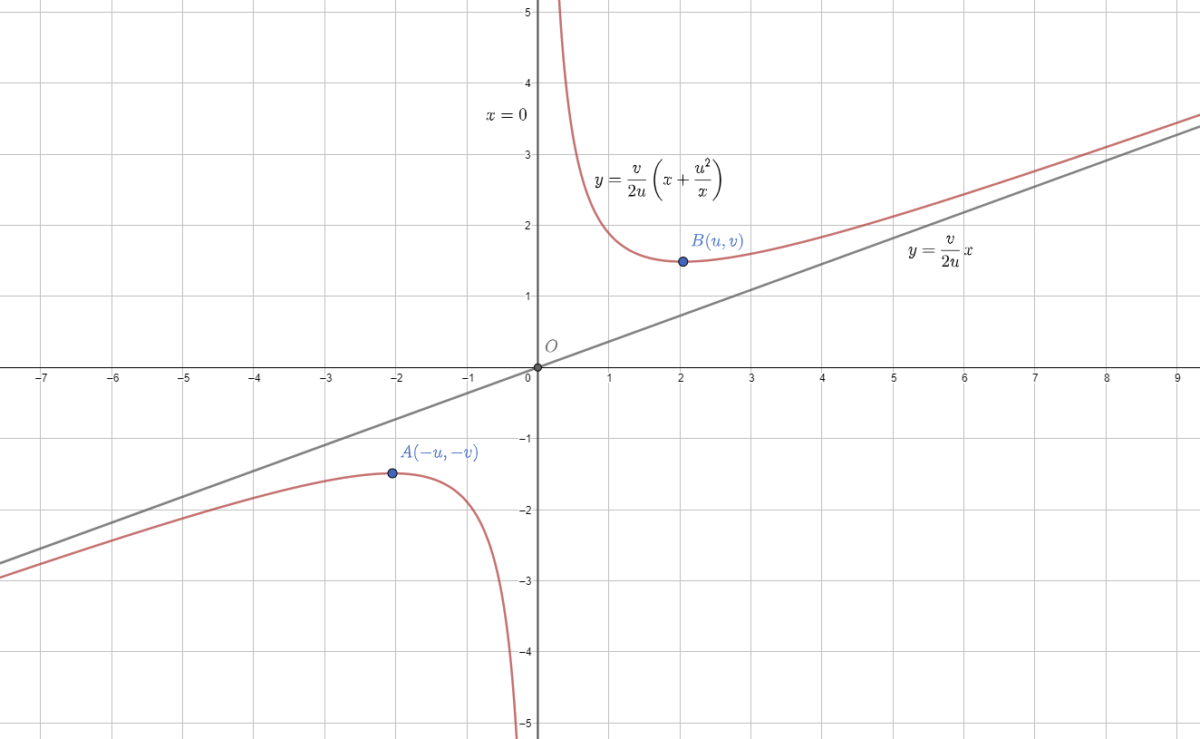# Visualize the vertexThis week’s Riddler Classic is a neat geometry problem about

Suppose you have two distinct points anywhere on the coordinate plane. If I tell you that a parabola with a vertical line of symmetry passes through those two points, where on the plane could that parabola’s vertex be?

Here is my solution:
[Show Solution]